# Compare

Compare fractions
$\frac{34}{3}$
and
$\frac{12}{4}$
. Which fraction of the lower?

Result

### Step-by-step explanation: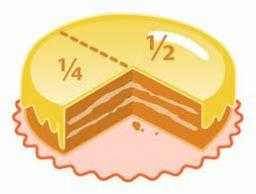Did you find an error or inaccuracy? Feel free to write us. Thank you!Tips to related online calculators
Need help to calculate sum, simplify or multiply fractions? Try our fraction calculator.

## Related math problems and questions:

• FractionFind for what x fraction (-4x -6)/(x) equals:
• Simplest form of a fractionWhich one of the following fraction after reducing in simplest form is not equal to 3/2? a) 15/20 b) 12/8 c) 27/18 d) 6/4
• Comparing and sortingArrange in descending order this fractions: 2/7, 7/10 & 1/2
• Sort fractions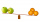Which of the following fractions is the largest? 29/36 5/6 7/9 3/4
• What fraction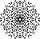What fraction of number 1 to 30 is prime?
• Equivalent fractions 2Write the equivalent multiplication expression. 2 1/6÷3/4
• Guess a fractionTom was asked to guess a fraction. The sum of 1/2 the numerator and 1/3 of its denominator is 30. If Tom subtracts 36 from its denominator, the fraction becomes 1/3. What is the fraction that Tom was asked to guess? (Leave your answer in simplest form)
• Camp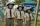In the camp are children. 1/2 went on a trip, 1/4 went to bathe, and 38 children remained in the room. How many children are in the camp?
• Fractions and mixed numerals(a) Convert the following mixed numbers to improper fractions. i. 3 5/8 ii. 7 7/6 (b) Convert the following improper fraction to a mixed number. i. 13/4 ii. 78/5 (c) Simplify these fractions to their lowest terms. i. 36/42 ii. 27/45 2. evaluate the follow
• Compare: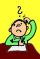What is bigger? ...
• Without 2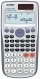Without multipying, tell whether the product 0.644 x 0.25 will be greater than 1 or less than 1? Explane how you know. Then find the product.
• FruitsAmy bought a basket of fruits 1/5 of them were apples,1/4 were oranges, and the rest were 33 bananas. How many fruits did she buy in all?
• How manyHow many integers are greater than 547/3 and less than 931/4?
• To improper fractionChange mixed number to improper fraction a) 1 2/15 b) -2 15/17
• Two fraction equation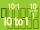Find d value of q in the equation 30/16=q/48.
• Two numbers and its productThe product of two numbers are 2/3. If on of them is 1/10, what is the other?
• Paper collecting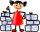At the paper collecting contest gathered Franta 2/9 ton, Karel 1/4 ton, and Patrick 19/36 tons of paper. Who has gathered the most and the least?